# PSAT Math : How to find the next term in an arithmetic sequence

## Example Questions

### Example Question #1 : How To Find The Next Term In An Arithmetic Sequence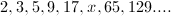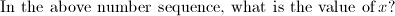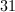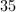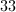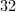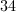Explanation:

Each term in the sequence is one less than twice the previous term.

So,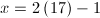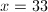### Example Question #2 : How To Find The Next Term In An Arithmetic Sequence

What is the next number in the following series: 0, 3, 8, 15, 24 . . . ?

32

35

41

37

40

35

Explanation:

The series is defined by n2 – 1 starting at n = 1. The sixth number in the series then equal to 62 – 1 = 35.

### Example Question #1 : How To Find The Next Term In An Arithmetic Sequence

A sequence of numbers is as follows: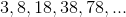What is the sum of the first seven numbers in the sequence?

719

1529

621

248

490

621

Explanation:

The pattern of the sequence is (x+1) * 2.

We have the first 5 terms, so we need terms 6 and 7:

(78+1) * 2 = 158

(158+1) * 2 = 318

3 + 8 + 18 +38 + 78 + 158 + 318 = 621

### Example Question #181 : Integers

Find the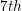term in the sequence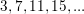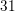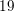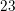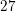Explanation:

Notice that in the sequenceeach term increases by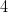.

It is always good strategy when attempting to find a pattern in a sequence to examine the difference between each term.

We continue the pattern to find:

The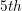term isThe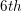term isTheterm isIt is useful to note that the sequence is defined by,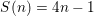where n is the number of any one term.

We can solve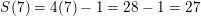to find theterm.